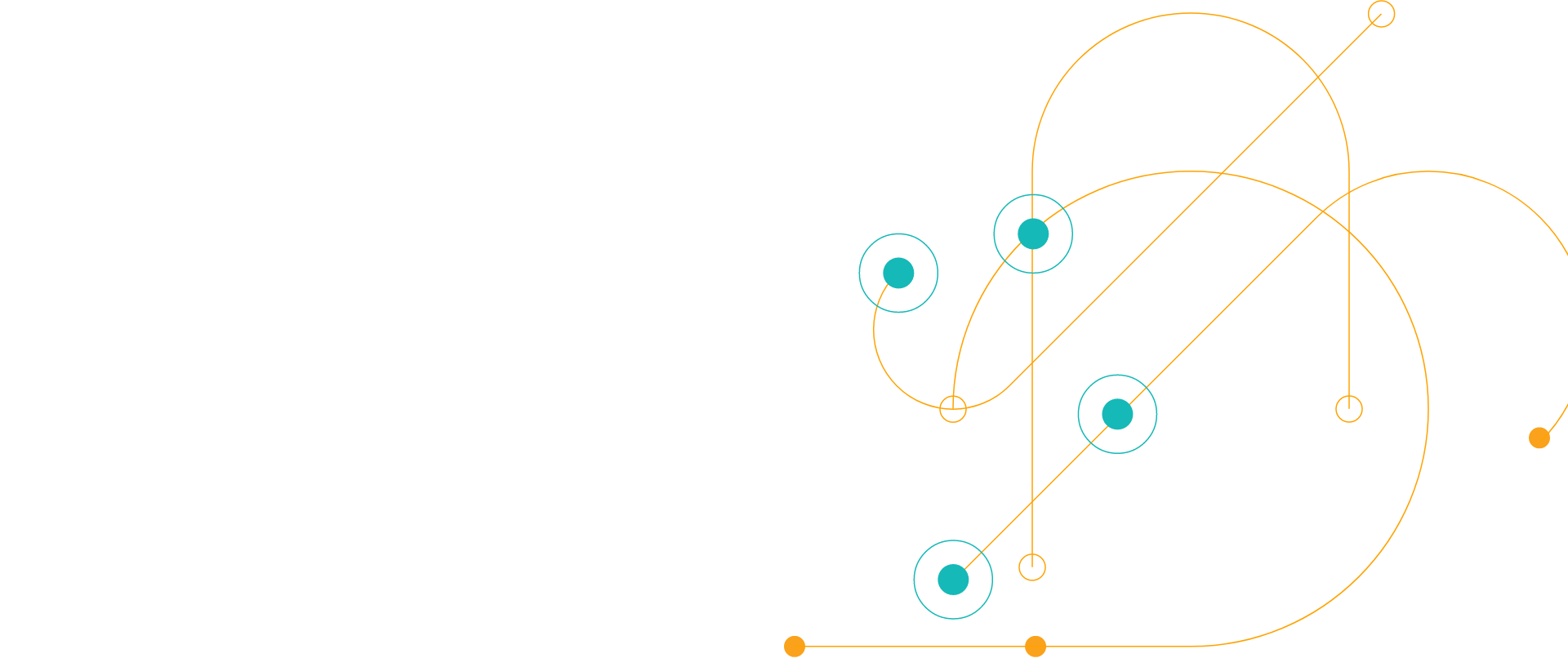# Embrace DevOps eBook

Your Guide to the DevOps Lifestyle

Back to eBooks

## Scale DevOps by Building a Coded Enterprise

DevOps is a practice at the center of organizational transformation. It requires collaboration across teams, the adoption of automation and consistent delivery paths for all applications.  Savvy companies have realized the way to scale DevOps is by building a coded enterprise. Collaboration through code enables groups with different languages and goals to work together.

Our Embrace DevOps eBook is filled with DevOps best practices that, when combined with a DevOps solution like Chef Enterprise Automation Stack, make it possible to collaborate via code and build better products with greater speed.

<div class='-tac -pb2'> <div class='svgi -c-magenta -mb1'> <svg xmlns='http://www.w3.org/2000/svg' width='178.099' height='129.473' viewBox='0 0 178.099 129.473'><defs><style>.a{fill:none;stroke:#d91683;stroke-miterlimit:10;stroke-width:2px;}</style></defs><g transform='translate(-964 -388)'><g transform='translate(2053.531 592.899)'><path class='a' d='M-1001.416-112.527h0a15.244,15.244,0,0,0,15.242-15.244v-7.543a2.33,2.33,0,0,1,2.332-2.33h1.15a7.628,7.628,0,0,1,7.628,7.627v17.49h12.3a7.469,7.469,0,0,1,7.469,7.469,7.481,7.481,0,0,1-.291,2.065l-5.254,18.267a7.47,7.47,0,0,1-7.178,5.4h-23.82'/><path class='a' d='M-1016.167-89.561v-22.586a5.337,5.337,0,0,1,5.337-5.339h4.075a5.339,5.339,0,0,1,5.339,5.339v36.721'/><circle class='a' cx='2.418' cy='2.418' r='2.418' transform='translate(-1027.341 -153.295)'/><circle class='a' cx='3.089' cy='3.089' r='3.089' transform='translate(-932.889 -108.485)'/><circle class='a' cx='3.089' cy='3.089' r='3.089' transform='translate(-1045.892 -148.25)'/><line class='a' transform='translate(-1032.363 -112.148)'/><line class='a' x1='7.549' transform='translate(-1024.031 -112.148)'/><line class='a' x1='11.31' transform='translate(-1027.341 -79.57)'/><line class='a' y1='30.648' transform='translate(-982.378 -199.62)'/><line class='a' y1='9.209' transform='translate(-987.339 -204.899)'/><line class='a' x2='14.429' transform='translate(-945.128 -133.443)'/><line class='a' x2='16.313' transform='translate(-1029.224 -133.443)'/><line class='a' x1='17.459' y1='17.459' transform='translate(-1024.252 -173.651)'/><line class='a' y1='17.459' x2='17.459' transform='translate(-954.858 -173.657)'/><line class='a' x2='15.442' transform='translate(-926.874 -133.443)'/><circle class='a' cx='5.045' cy='5.045' r='5.045' transform='translate(-924.198 -158.341)'/><circle class='a' cx='3.93' cy='3.93' r='3.93' transform='translate(-956.811 -203.551)'/><path class='a' d='M-1002.58-130.114a21.3,21.3,0,0,1,21.3-21.295,21.3,21.3,0,0,1,21.295,21.295'/><path class='a' d='M-1007.275-130.674a25.99,25.99,0,0,1,25.99-25.99,25.99,25.99,0,0,1,25.99,25.99'/><path class='a' d='M-1022.911-112.861a32.811,32.811,0,0,1-32.81,32.81,32.811,32.811,0,0,1-32.81-32.81'/><circle class='a' cx='25.308' cy='25.308' r='25.308' transform='translate(-1081.029 -137.456)'/><path class='a' d='M-1065.55-107.007l9.829,7.8,9.829-7.8'/><line class='a' y2='25.892' transform='translate(-1055.721 -125.094)'/><circle class='a' cx='0.565' cy='0.565' r='0.565' transform='translate(-969.36 -164.564)'/><circle class='a' cx='0.565' cy='0.565' r='0.565' transform='translate(-945.692 -110.858)'/><line class='a' x1='3.557' y2='3.557' transform='translate(-939.276 -184.213)'/><line class='a' x1='9.946' y2='9.946' transform='translate(-934.773 -186.311)'/><line class='a' x1='9.946' y2='9.946' transform='translate(-937.266 -173.945)'/><line class='a' x1='9.946' y1='9.946' transform='translate(-1039.929 -186.311)'/><line class='a' x1='9.946' y1='9.946' transform='translate(-1037.436 -173.945)'/><circle class='a' cx='2.605' cy='2.605' r='2.605' transform='translate(-1012.516 -82.175)'/></g></g></svg> </div> <h3>Thank You</h3> <p>If your download hasn't started in 5 seconds please</p> <p><a data-sf-ec-immutable='' href='https://d1l5pp53ux74mz.cloudfront.net/docs/default-source/ebook/embracedevops.pdf?sfvrsn=ae0a7c00_2' class='-i-next' target='_blank' rel='nofollow'>Click here to download </a></p> </div> <script>document.getElementsByClassName('js-form-title').style.display='none';var win=window.open('https://d1l5pp53ux74mz.cloudfront.net/docs/default-source/ebook/embracedevops.pdf?sfvrsn=ae0a7c00_2', '_blank');win.focus();</script><script>if (typeof (window.dataLayer) !== 'undefined') { window.dataLayer = window.dataLayer || []; dataLayer.push({'event': 'custom.successMessageShown'}); } </script>